# IBPS RRB 2018 Prelims : Reasoning – Day 16

Dear Bankersdaily Aspirants,

IBPS RRB PO & Office Assistant 2018 Preliminary Examination starts from 11th August, 2018. The IBPS RRB Exam 2018 is one of the most expected exam of the year. The reason for the consummate expectation is different from that of the others.

The number of candidates attending the IBPS RRB PO & Office Assistant is high when compared to the other exams like SBI & Insurance Exams. The other important factor is that , no English section is there in the preliminary examination of the IBPS RRB PO & Assistant Exams. This is really a boost to the candidates as many of the aspirants couldn’t get the requisite marks in the English section and this exam is the best bet for the aspirants.

Since only few days are left for the IBPS RRB PO & Assistant Exam 2018 Preliminary Exam , we will be providing daily 20 Questions in Quantitative Aptitude & Reasoning Section to evoke the preparation mode.

Aspirants can answer the Questions by commenting the answers for the Questions in the comments section. We will be providing the answers for the Questions @ 6:00 P.M everyday.

## IBPS RRB PO & Assistant 2018 – Prelims Exam – Day 16

Topic : Reasoning Ability

Total Number of Questions : 20

Total Time : 15 minutes

D.1-5) In each question, relationship between different elements is shown in the statements. The statements are followed by conclusions. Study the conclusions based on the given statements and select the appropriate answer.

Q.1) Statements:

T<I<P≤C=N≥L>K; F≥A>C<B≤E

Conclusion:

I. I>A

II. L<E

III. L≤F

a) Only I follows

b) Only II follows

c) Only III follows

d) Only I and III follow

e) None of these

Q.2) Statement:

P<Q=N<C≤K; M>J>N<D; L<R≤C<U

Conclusion:

I. P<U

II. M>K

III. D>L

a) Only II follows

b) None follows

c) Only III follows

d) Only I and III follow

e) None of these

Q.3) Statement:

E>U=C<D<H≤O; D=M>P≥Z; I<Z<X≤L

Conclusion:

I. O>I

II. E>L

III. L≥E

a) Only I follows

b) Either II or III follows

c) Only I and either II or III follow

d) None follows

e) None of these

Q.4) Statement:

K>O>H>P=N≥A; B<D=E≤A; O>L=Q

Conclusion:

I. P>E

II. Q>K

III. P=E

a) Only II and either I or III follow

b) None follows

c) Only III follows

d) Only I and III follow

e) None of these

Q.5) Statement:

I>L>P≥K=M<S<J; O>L<T; M>A=G

Conclusion:

I. G<L

II. T>M

III. J>A

a) Only II and III follow

b) All I, II and III follow

c) Only I and III follow

d) Only I and III follow

e) None of these

D.6-10) In each question below are statements followed by conclusion. You have to take the given statements to be true even if they seem to be at variance with commonly known facts. Read all the conclusions and then decide which of the given conclusions logically follows from the given statements disregarding commonly known facts.

Q.6) Statements:

All Highways are Bye pass.

All Bye pass are Bridges.

Some Bridges are Canal.

Conclusions:

I. All Bridges are Highways

II. Some Canal is not Road

a) Only I follows

b) Only II follows

c) Both I and II follow

d) Either I or II follows

e) Neither I nor II follows

Q.7) Statements:

All Televisions are LED.

No LED is a LCD.

No LCD is a CRT.

All CRT are Monitors.

Conclusions:

I. Some LEDs are CRT

II. No CRT is a LED

a) Only I follows

b) Only II follows

c) Both I and II follow

d) Either I or II follows

e) Neither I nor II follows

Q.8) Statements:

All Monkeys are Birds.

All Birds are Animals.

Some Animals are Mammals.

No Mammal is an insect.

Conclusions:

I. All animal can never be insect

II. All birds can never be insect

a) Only I follows

b) Only II follows

c) Both I and II follow

d) Either I or II follows

e) Neither I nor II follows

Q.9) Statements:

All Busses are Tempo.

Some Tempo’s are Trains.

All Trains are Aeroplanes.

No Aeroplane is a Helicopter.

Conclusions:

I. Some Trains are Helicopter is a possibility

II. All Busses are Train is a Possibility

a) Only I follows

b) Only II follows

c) Both I and II follow

d) Either I or II follows

e) Neither I nor II follows

Q.10) Statements:

All Notes are Book.

All Books are Paper.

No Paper is a Pen.

No Pen is a Laptop.

Conclusions:

I. All Laptop being paper is a possibility

II. Some Papers are Notes

a) Only I follows

b) Only II follows

c) Both I and II follow

d) Either I or II follows

e) Neither I nor II follows

D.11-15) Study the following series of alpha-numeric-symbol combination and answer the following questions.

H 4 * 5 K J % 2 T E 1 # S 6 £ Q C \$ F 8 M A @ 9 L & 3 G V 7 B W

Q.11) How many letters are there in the above arrangement each which is immediately preceded by a number but not immediately followed by a symbol?

a) None

b) One

c) Three

d) Five

e) None of these

Q.12)Which of the following is the fifth to the left of the tenth from the right end of the above arrangement?

a) @

b) F

c) \$

d) C

e) None of these

Q.13) Four of the following five are alike in a certain way based on the arrangement and hence form a group. Which one of the following does not belong to the group?

a) T # £

b) M 9 3

c) Q \$ A

d) L G B

e) % E S

Q.14) If all the letters and numbers are dropped from the above arrangement then, which of the following will be the sixth from the right end of the above arrangement?

a) @

b) %

c) #

d) \$

e) None of these

Q.15) How many such consonants are there in the above arrangement, each of which is immediately preceded by a number and immediately followed by a symbol?

a) None

b) One

c) Two

d) Three

e) More than three

D.16-18) Study the following information carefully and answer the questions given below.

M, O, P, Q, R, S, T, U and V are members of a family. R is son-in-law of P. Q is maternal aunt of S. Q is daughter-in-law of V. P is grandmother of S. M has two daughter only. U is daughter of V. T is brother-in-law of Q. S is son of O. U has only one brother. There are three married couple in the family.

Q.16) How is U related to Q?

a) Sister

b) Brother

c) Sister-in-law

d) Father

e) None of these

Q.17) How is M related to S?

a) Grandson

b) Grand father

c) Brother

d) Father

e) None of these

Q.18) If V is father of R then how many females are in the family?

a) One

b) Four

c) Three

d) Five

e) Cannot be determined

D.19-20) Study the following information carefully and answer the questions given below.

Point A is 10 m west of Point B. Point F is east of Point E. Point D is 9 m south of Point E. Point C is 15 m west of Point D. Point B is 24 m north of Point C. The distance between Point E and F is 3 m less than the distance between Point C and D. Point G is south of Point F.

Q.19) What is the sum of shortest distance between point A and C and Point D and F?

a) 35m

b) 34m

c) 38m

d) 41m

e) Cannot be determined

Q.20) Point G is in which direction with respect to Point C?

a) West

b) South west

c) North east

d) East

e) Cannot be determined

Explanations

Q.1) (b)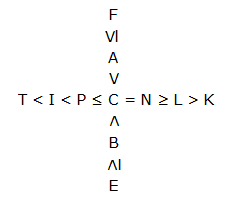I. I>A (False)

II. L<E (True)

III. L≤F (False)

Q.2) (e)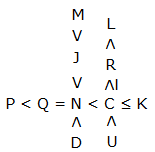I. P<U (True)

II. M>K (False)

III. D>L (False)

Q.3) (c)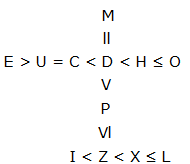I. O>I (True)

II. E>L (False) (either or)

III. L≥E (False) (either or)

Q.4) (e)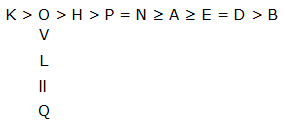I. P>E (False) either ‘or’

II. Q>K (False)

III. P=E (False) either ‘or’

Q.5) (b)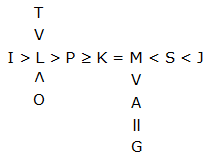I. G<L (True)

II. T>M (True)

III. J>A (True)

Q.6) (e)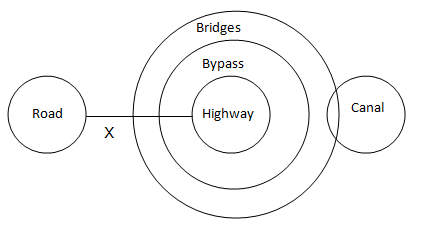I. False

II. False

Q.7) (d)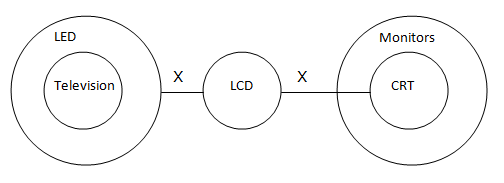I. False (either or)

II. False (either or)

Q.8) (a)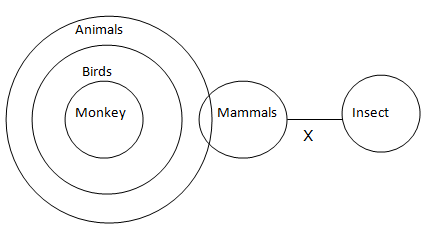I. True

II. False

Q.9) (b)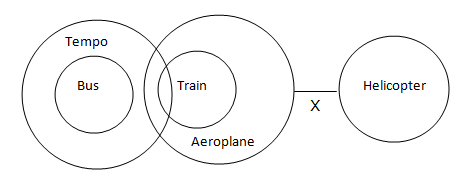I. False

II. True

Q.10) (c)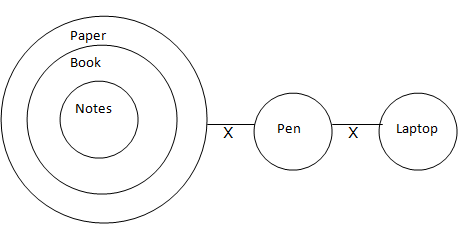I. True

II. True

Q.11) (d)

5KJ, 2TE, 8MA, 3GV, 7BW.

Q.12) (c)

5th to the left + 10th to the right = 15th from the right

\$

Q.13) (c)

Except ‘Q \$ A’ there are two elements in between first and second elements as well as second and third elements.

Q.14) (b)

* % # £ \$ @ &

Q.15) (b)

9 L &

D.16-10)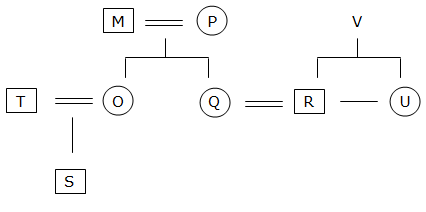Q.16) (c)

Q.17) (b)

Q.18) (b)

Q.19) (d)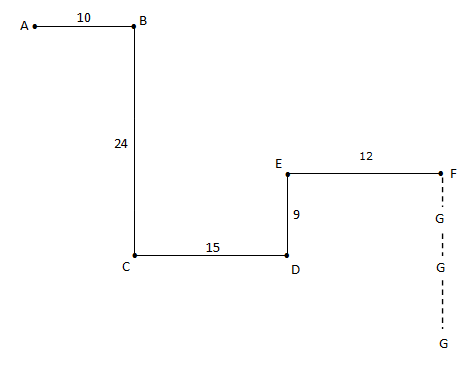AC = 26

DF = 15

= 41

Q.20) (e)We will post this Practice Questions – Daily till the start of the IBPS RRB PO & Assistant (Regularly)

Quantitative Aptitude @ 3:00 P.M

Reasoning Ability -@ 5:00 P.M

 QUANTITATIVE APTITUDE REASONING DAY 1 DAY 1 DAY 2 DAY 2 DAY 3 DAY 3 DAY 4 DAY 4 DAY 5 DAY 5 DAY 6 DAY 6 DAY 7 DAY 7 DAY 8 DAY 8 DAY 9 DAY 9 DAY 10 DAY 10 DAY 11 DAY 12 DAY 12 DAY 13 DAY 13 DAY 14 DAY 15 DAY 15

Attend GEEK TEST – SOLVE 10 Daily and enhance your preparation to the next level. Attend the Daily Tests with the Timer and improve your solving ability.

Also Please attend this BLUE WHALE QUESTION (which is a combination of many topics and combined into a single one). This is a One Stop Solution for all your Miscellaneous needs.

## BLUE WHALE CHALLENGE QUESTIONS

Get the FULL LENGTH MOCK TEST SERIES for SBI PO Mains 2018 , SBI CLERK Mains 2018 & BOB PO Mains 2018 from our Bankersdaily Store.